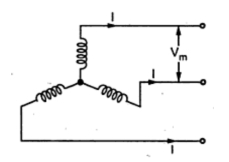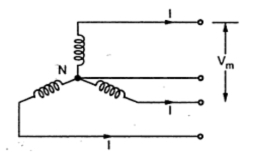### Comparison of Volume of Copper in Underground System

In case of underground system, the maximum stress exists between the line conductors. Hence the various assumptions for such comparison are,
1. The maximum voltage (Vm), between the conductors is same.
2. The power (P) transmitted in all the systems is same.
3. The distance ( l  ) over which the power is transmitted is same.
4. The copper losses (w) are same in all the systems.
1.1 Two Wire D.C. System
The system is represented in the Fig. 1.Fig. 1

The voltage between the two conductors is Vm. It can be seen that there are no changes in the condition which we have discussed for two wire D.C. system for overhead system earlier in (previous post section 1.1).

This is the base of comparison of other systems,
1.2 Two Wire D.C. System With Midpoint Earthed
The system is shown in the Fig. Fig. 2.Fig. 2

it can be noted that the maximum voltage Vm exists between the two lines.

Hence the total volume of copper required is,

Thus the volume of copper required in this system is same as that required for two wire d.c. system.
1.3 Three Wire D.C. System
The system is represented in the Fig. 3.Fig. 3

Again the voltage between the two lines is maximum which is Vm.

Assume balanced load so there is no current through the neutral and there are no copper losses in neutral. So total copper loss is,

where  A = area of cross-section of each line conductor.

Let area of cross-section of neutral wire is half of the area of cross-section of each conductor i.e. 0.5 A.
Hence the total volume of copper required is

Thus the volume of copper required for this system is 1.25 times that that required for 2 wire d.c. system. So 25% more copper is required in this system.
1.4 Three Phase Three Wire A.C. System
Consider a star connected three phase three wire a.c. system as shown in the Fig. 4. Note that the voltage between the line conductors is Vm. Thus the line voltage is Vm.Fig. 4

The r.m.s. value of the phase voltage is,

The power transmitted per phase is,

Let cosΦ be the load power factor.

Hence the volume of copper required is,

Thus the volume of copper required in this system depends on the load power factor and it is 1.5/cos2Φ times that that required for two wire d.c. system.
The resultant remains same whether the system is star or delta connected.
1.5 Three Phase Four Wire A.C. System
The system is shown in the Fig. 5. The neutral is available for the load connection. As the load is assumed to be balanced, the neutral current is zero and the copper losses in neutral are zero. Note that the voltage between the lines is Vm.Fig. 5

Hence the r.m.s. value of the phase voltage is,

The power per phase is given by,

This is same as obtained for three phase three wire system. The total copper loss is,

Let the cross-section of the neutral wire be half of the cross-section of each line conductor i.e. 0.5 A.
Hence the total volume of copper required is

Thus the volume of copper required in this system is 1.75/cos2Φ times the volume of copper required for two wire d.c. system.